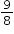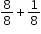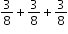# MA.4.FR.2.1

Decompose a fraction, including mixed numbers and fractions greater than one, into a sum of fractions with the same denominator in multiple ways. Demonstrate each decomposition with objects, drawings and equations.

### Examplescan be decomposed asor as.

### Clarifications

Clarification 1: Denominators are limited to 2, 3, 4, 5, 6, 8, 10, 12, 16 and 100.
General Information
Subject Area: Mathematics (B.E.S.T.)
Strand: Fractions
Status: State Board Approved

## Related Courses

This benchmark is part of these courses.
5012060: Mathematics - Grade Four (Specifically in versions: 2014 - 2015, 2015 - 2022, 2022 and beyond (current))
7712050: Access Mathematics Grade 4 (Specifically in versions: 2014 - 2015, 2015 - 2018, 2018 - 2022, 2022 and beyond (current))
5012055: Grade 3 Accelerated Mathematics (Specifically in versions: 2019 - 2022, 2022 and beyond (current))
5012015: Foundational Skills in Mathematics 3-5 (Specifically in versions: 2019 - 2022, 2022 and beyond (current))

## Related Access Points

Alternate version of this benchmark for students with significant cognitive disabilities.
MA.4.FR.2.AP.1: Decompose a fraction less than one into a sum of unit fractions with the same denominator (e.g., 3/4 = 1/4 + 1/4 + 1/4). Denominators limited to 2, 3, 4, 6, 8 or 10. Demonstrate each decomposition with objects, drawings or equations.

## Related Resources

Vetted resources educators can use to teach the concepts and skills in this benchmark.

## Formative Assessment

Decomposing Three-Fifths:

Students are asked to use a visual fraction model to decompose three-fifths in two different ways.

Type: Formative Assessment

## Image/Photograph

Clipart ETC Fractions:

Illustrations that can be used for teaching and demonstrating fractions. Fractional representations are modeled in wedges of circles ("pieces of pie") and parts of polygons. There are also clipart images of numerical fractions, both proper and improper, from halves to twelfths. Fraction charts and fraction strips found in this collection can be used as manipulatives and are ready to print for classroom use.

Type: Image/Photograph

## Lesson Plans

Relay Races:

In this lesson, students solve word problems related to races to determine addends of fractions with like denominators that sum to a fraction that is less than or equal to one and has the same denominator as the addends. The focus is on addition, decomposing a fraction into a sum of fractions in more than one way, drawing linear models, and writing equations to represent the problems.

Type: Lesson Plan

Decomposing Fractions:

Using circle fraction manipulative, students will decompose fractions to discover adding fractions with like denominators.

Type: Lesson Plan

## Original Student Tutorial

The Leftover Dessert Dilemma:

Learn how to decompose a fraction into a sum of fractions with common denominators with this interactive tutorial.

Type: Original Student Tutorial

Making 22 Seventeenths in Different Ways:

This task is a straightforward task related to adding fractions with the same denominator. The main purpose is to emphasize that there are many ways to decompose a fraction as a sum of fractions.

Expanded Fractions and Decimals:

The purpose of this task is for students to show they understand the connection between fraction and decimal notation by writing the same numbers both ways. Comparing and contrasting the two solutions shown below shows why decimal notation can be confusing. The first solution shows the briefest way to represent each number, and the second solution makes all the zeros explicit.

Writing a Mixed Number as an Equivalent Fraction:

The purpose of this task is to help students understand and articulate the reasons for the steps in the usual algorithm for converting a mixed number into an equivalent fraction. Step two shows that the algorithm is merely a shortcut for finding a common denominator between two fractions. This concept is an important precursor to adding mixed numbers and fractions with like denominators and as such, step two should be a point of emphasis. This task is appropriate for either instruction or formative assessment.

## Virtual Manipulative

Fraction Game:

This virtual manipulative allows individual students to work with fraction relationships. (There is also a link to a two-player version.)

Type: Virtual Manipulative

## MFAS Formative Assessments

Decomposing Three-Fifths:

Students are asked to use a visual fraction model to decompose three-fifths in two different ways.

## Original Student Tutorials Mathematics - Grades K-5

The Leftover Dessert Dilemma:

Learn how to decompose a fraction into a sum of fractions with common denominators with this interactive tutorial.

## Student Resources

Vetted resources students can use to learn the concepts and skills in this benchmark.

## Original Student Tutorial

The Leftover Dessert Dilemma:

Learn how to decompose a fraction into a sum of fractions with common denominators with this interactive tutorial.

Type: Original Student Tutorial

Making 22 Seventeenths in Different Ways:

This task is a straightforward task related to adding fractions with the same denominator. The main purpose is to emphasize that there are many ways to decompose a fraction as a sum of fractions.

Expanded Fractions and Decimals:

The purpose of this task is for students to show they understand the connection between fraction and decimal notation by writing the same numbers both ways. Comparing and contrasting the two solutions shown below shows why decimal notation can be confusing. The first solution shows the briefest way to represent each number, and the second solution makes all the zeros explicit.

Writing a Mixed Number as an Equivalent Fraction:

The purpose of this task is to help students understand and articulate the reasons for the steps in the usual algorithm for converting a mixed number into an equivalent fraction. Step two shows that the algorithm is merely a shortcut for finding a common denominator between two fractions. This concept is an important precursor to adding mixed numbers and fractions with like denominators and as such, step two should be a point of emphasis. This task is appropriate for either instruction or formative assessment.

## Virtual Manipulative

Fraction Game:

This virtual manipulative allows individual students to work with fraction relationships. (There is also a link to a two-player version.)

Type: Virtual Manipulative

## Parent Resources

Vetted resources caregivers can use to help students learn the concepts and skills in this benchmark.

## Image/Photograph

Clipart ETC Fractions:

Illustrations that can be used for teaching and demonstrating fractions. Fractional representations are modeled in wedges of circles ("pieces of pie") and parts of polygons. There are also clipart images of numerical fractions, both proper and improper, from halves to twelfths. Fraction charts and fraction strips found in this collection can be used as manipulatives and are ready to print for classroom use.

Type: Image/Photograph

Making 22 Seventeenths in Different Ways:

This task is a straightforward task related to adding fractions with the same denominator. The main purpose is to emphasize that there are many ways to decompose a fraction as a sum of fractions.